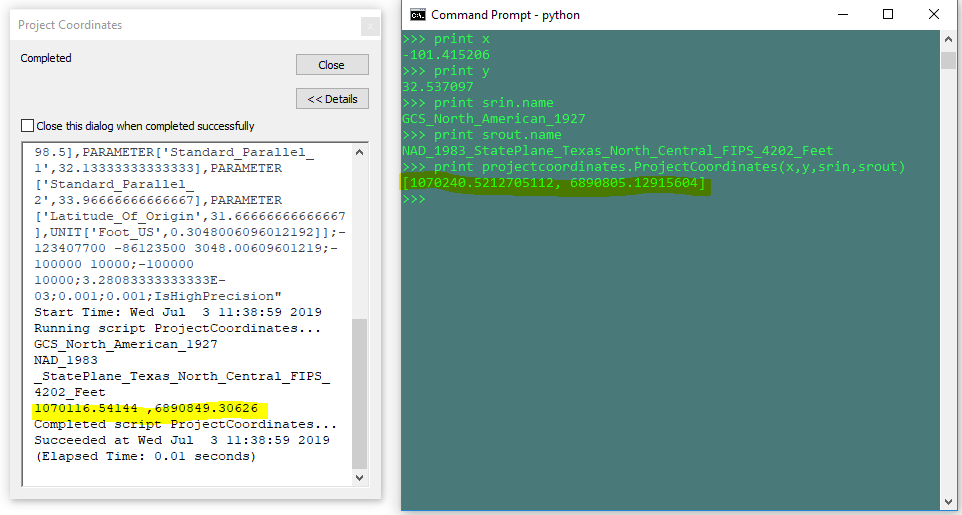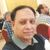# Using 'projectAs()' Point Geometry Function Via Python Function in Command Prompt

2558
5
07-03-2019 09:41 AMNew Contributor III

So, i have a python function defined that projects coordinates using the 'projectAs()' point geometry function. When i call this function in a script from a toolbox it gives different results vs calling it in a python command prompt window. See below python function below:

``````def ProjectCoordinates(x,y,inputcrs,outputcrs):
import arcpy
point = arcpy.PointGeometry(arcpy.Point(x,y),inputcrs)
projectedpoint = point.projectAs(outputcrs)
projectedcoordinates = [projectedpoint.firstPoint.X,projectedpoint.firstPoint.Y]

return projectedcoordinates‍‍‍‍‍‍‍``````

Full Toolbox Script:

``````def ProjectCoordinates(x,y,inputcrs,outputcrs):
import arcpy
point = arcpy.PointGeometry(arcpy.Point(x,y),inputcrs)
projectedpoint = point.projectAs(outputcrs)
projectedcoordinates = [projectedpoint.firstPoint.X,projectedpoint.firstPoint.Y]

return projectedcoordinates

import arcpy

xin = arcpy.GetParameterAsText(0)
yin = arcpy.GetParameterAsText(1)

srin = arcpy.GetParameter(2)
srout = arcpy.GetParameter(3)

xout,yout = ProjectCoordinates(xin,yin,srin,srout)

arcpy.AddMessage(str(xout) + " ," + str(yout))

‍‍‍‍‍‍‍‍‍‍‍‍‍‍‍‍‍‍‍‍‍‍``````

Results of Each:Can anyone replicate this? Does anyone have any ideas why this is happening?

Also, if it helps, I know from surveyed data that the toolbox script output is correct and the command prompt output is incorrect.

Tags (4)
1 Solution

Accepted Solutionsby
New Contributor II

Well, I have struggled a bit without any luck, I was Passing the name of Spatial Reference System it did not work. Problem is displayed name in the Spatial Reference System is Different than the name used in this function projectAs().

I solved it by passing the Spatial Reference Object for example I created outPut coordinate System using

target_Ref = arcpy.SpatialReference(4326)

point.projectAs(target_Ref)

it worked fine

5 RepliesbyMVP Legendary Contributor

datum shift... I am thinking that the plain script doesn't deal with it, but the toolbox script is 'smart' enough to detect it.

Worth a check anywaybyMVP Regular Contributor

I did some testing and can confirm that the tool script and code run in the Python window produce different results.  I believe this is due to an automatic transformation that is occurring when the tool is being run.  The GCS NA 1927 coordinates appear to be transformed into GCS NA 1983 using NAD 1927 To NAD 1983 NADCON.

As an experiment I created two layers: the Texas State Plane that you are working with, and a GCS NA 1927.  The state plane was populated with two points located at the positions you highlighted in your results.  The GCS 1927 was populated with one point using the lon/lat in your script.  The state plane was placed in a new map.  When adding the GCS 1927 layer a transformation dialog was presented.  If no transformation was selected, the GCS 1927 point was placed over the state plane point located at 1070240.5212705112, 6890805.12915604.  If the NAD 1297 To NAD 1983 NADCON transformation was used, the GCS 1927 point was located over the state plane point located at 1070116.54144, 6890849.30626.

While experimenting, I noticed that the Add Geometry Attributes tool also automatically included the transformation.

I suppose the next question is:  Can the transformation be added to the projectAs() function?byMVP Legendary Contributor

Of course!  The help file topic has the required info.  This is for the generic Geometry Class... it trickles down to the other classes

 projectAs (spatial_reference, {transformation_name}) Projects a geometry and optionally applies a geotransformation.To project, the geometry needs to have a spatial reference, and not have an UnknownCoordinateSystem. The new spatial reference system passed to the method defines the output coordinate system. If either spatial reference is unknown the coordinates will not be changed. The Z- and measure values are not changed by the ProjectAs method.byMVP Regular Contributor

I got this to work in 10.6:

``````import arcpy

x = -101.415206
y = 32.537097

print ptGeometry.firstPoint.X, ptGeometry.firstPoint.Y
# result: 1070116.54144 6890849.30626
‍‍‍‍‍‍‍‍‍``````

It seems that when you use a "transformation_name", the associated "spatial_reference" must be a name (not a factory code).  This document ArcGIS 10.7.1 and ArcGIS Pro 2.4 Geographic and Vertical Transformation Tables was helpful.by
New Contributor II

Well, I have struggled a bit without any luck, I was Passing the name of Spatial Reference System it did not work. Problem is displayed name in the Spatial Reference System is Different than the name used in this function projectAs().

I solved it by passing the Spatial Reference Object for example I created outPut coordinate System using

target_Ref = arcpy.SpatialReference(4326)

point.projectAs(target_Ref)

it worked fine